Courses

# RD Sharma Solutions -Ex-15.2, Areas Of Parallelograms And Triangles, Class 9, Maths Class 9 Notes | EduRev

## Class 9 : RD Sharma Solutions -Ex-15.2, Areas Of Parallelograms And Triangles, Class 9, Maths Class 9 Notes | EduRev

The document RD Sharma Solutions -Ex-15.2, Areas Of Parallelograms And Triangles, Class 9, Maths Class 9 Notes | EduRev is a part of the Class 9 Course RD Sharma Solutions for Class 9 Mathematics.
All you need of Class 9 at this link: Class 9

Q 1. If figure, ABCD is a parallelogram, AE ⊥ DC and CF ⊥ AD. If AB = 16 cm, AE = 8 cm, and CF = 10 cm, Find AD.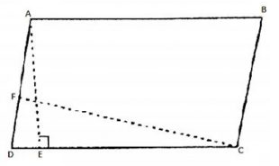Solution:

Given that,

In parallelogram ABCD, CD = AB = 16 cm                                [∵ Opposite side of a parallelogramare equal]

We know that,

Area of parallelogram = Base × Corresponding altitude

Area of parallelogram ABCD = CD × AE = AD × CF

16 cm × cm = AD × 10 cm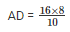cm = 12.8 cm

Thus, The length of AD is 12.8 cm.

Q 2. In Q 1, if AD = 6 cm, CF = 10 cm, and AE = 8 cm, Find AB.

Solution:

We know that,

Area of a parallelogram ABCD = AD × CF ⋅⋅⋅⋅⋅⋅⋅⋅⋅(1)

Again area of parallelogram ABCD = CD × AE⋅⋅⋅⋅⋅⋅⋅⋅⋅(2)

Compare equation(1) and equation(2)

AD × CF   = CD × AE

⇒6×10 = D×8

⇒D =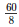= 7.5 cm

∴ AB = DC = 7.5cm                                     [∵Opposite side of a parallelogramare equal]

Q 3. Let ABCD be a parallelogram of area 124 cm. If E and F are the mid-points of sides AB and CD respectively, then find the area of parallelogram AEFD.

Solution:

Given,

Area of a parallelogram ABCD = 124 cm2

Construction: Draw AP⊥DC

Proof:-

Area of a parallelogram AFED = DF × AP  ⋅⋅⋅⋅⋅⋅⋅⋅⋅(1)

And  area of parallelogram EBCF = FC × AP⋅⋅⋅⋅⋅⋅⋅⋅⋅(2)

And DF = FC  ⋅⋅⋅⋅⋅⋅⋅⋅⋅(3)                                               [FisthemidpointofDC]

Compare equation (1), (2)  and (3)

Area of parallelogram AEFD = Area of parallelogram EBCF

∴ Area of parallelogram AEFD =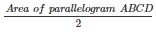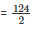=  62cm2

Q 4. If ABCD is a parallelogram, then prove that

Ar (ΔABD)  = Ar (ΔBCD)  = Ar (ΔABC)  = Ar (ΔACD)  =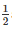Ar(//gmABCD).

Solution:

Given:-

ABCD is a parallelogram,

To prove : – Ar (ΔABD)  = Ar (ΔBCD)  = Ar (ΔABC)  = Ar (ΔACD)  =Ar(//gmABCD).

Proof:- We know that diagonal of a parallelogram divides it into two equilaterals .

Since, AC is the diagonal.

Then,  Ar (ΔABC)  = Ar (ΔACD)  =Ar(//gmABCD) ⋅⋅⋅⋅⋅⋅⋅⋅⋅(1)

Since, BD is the diagonal.

Then ,  Ar (ΔABD)  = Ar (ΔBCD)  =Ar(//gmABCD) ⋅⋅⋅⋅⋅⋅⋅⋅⋅(2)

Compare equation (1) and (2)

∴  Ar (ΔABC)  = Ar (ΔACD)  = Ar (ΔABD)  = Ar (ΔBCD)  =Ar(//gmABCD)..

Offer running on EduRev: Apply code STAYHOME200 to get INR 200 off on our premium plan EduRev Infinity!

91 docs

,

,

,

,

,

,

,

,

,

,

,

,

,

,

,

,

,

,

,

,

,

,

,

,

,

,

,

,

,

,

;